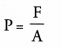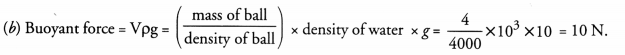## NCERT Exemplar Solutions for Class 9 Science Chapter 16 Floatation

These Solutions are part of NCERT Exemplar Solutions for Class 9 Science . Here we have given NCERT Exemplar Solutions for Class 9 Science Chapter 16 Floatation

MULTIPLE CHOICE QUESTIONS

Question 1.
An object is put one by one in three liquids having different densities. The object floats with 1/9, 2/11 and 3/7 parts of their volumes outside the liquid surface in liquids of densities d1, d2 and d3 respectively. Which of the following statement is correct ?
(a) d1 > d> d3
(b) d1 > d< d3
(c) d< d> d3
(d) d1 < d< d3
(d). Upthrust due to liquid on an object is directly proportional to the density of the liquid.

More Resources

Question 2.
An obj ect weighs 10 N in air. When immersed fully in water, it weighs only 8 N. The weight of the liquid displaced by the object will be
(a) 2 N
(b) 8 N
(c) 10 N
(d) 12 N.
(a). Explanation : Weight of liquid displaced by an object = Weight of object in air – weight in liquid.

Question 3.
A girl stands on a box having 60 cm length, 40 cm breadth and 20 cm width in three ways. In which of the following cases, pressure exerted by the brick will be
(a) maximum when length and breadth form the base
(b) maximum when breadth and width form the base
(c) maximum when width and length form the base
(d) the same in all the above three cases.
(b). Explanation :